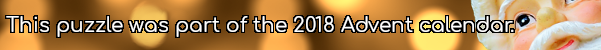mscroggs.co.uk
mscroggs.co.uksubscribe

# Puzzles

## Not Roman numerals

The letters $$I$$, $$V$$ and $$X$$ each represent a different digit from 1 to 9. If
$$VI\times X=VVV,$$
what are $$I$$, $$V$$ and $$X$$?

## 22 DecemberIn base 2, 1/24 is 0.0000101010101010101010101010...
In base 3, 1/24 is 0.0010101010101010101010101010...
In base 4, 1/24 is 0.0022222222222222222222222222...
In base 5, 1/24 is 0.0101010101010101010101010101...
In base 6, 1/24 is 0.013.
Therefore base 6 is the lowest base in which 1/24 has a finite number of digits.
Today's number is the smallest base in which 1/10890 has a finite number of digits.
Note: 1/24 always represents 1 divided by twenty-four (ie the 24 is written in decimal).

## 15 DecemberToday's number is smallest three digit palindrome whose digits are all non-zero, and that is not divisible by any of its digits.

## 9 DecemberToday's number is the number of numbers between 10 and 1,000 that contain no 0, 1, 2 or 3.

## 4 DecemberToday's number is the number of 0s that 611! (611×610×...×2×1) ends in.

## Square and cube endings

Source: UKMT 2011 Senior Kangaroo
How many positive two-digit numbers are there whose square and cube both end in the same digit?

## Digitless factor

Ted thinks of a three-digit number. He removes one of its digits to make a two-digit number.
Ted notices that his three-digit number is exactly 37 times his two-digit number. What was Ted's three-digit number?

## Backwards fours

If A, B, C, D and E are all unique digits, what values would work with the following equation?
$$ABCCDE\times 4 = EDCCBA$$

## Archive

Show me a random puzzle
▼ show ▼
© Matthew Scroggs 2019